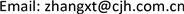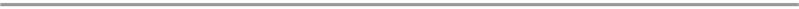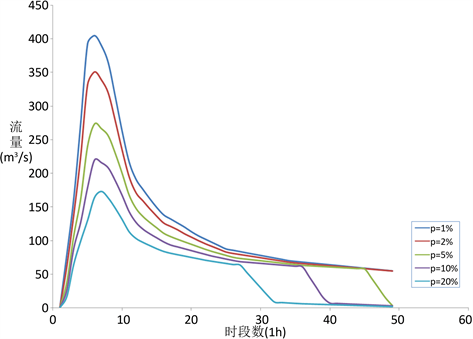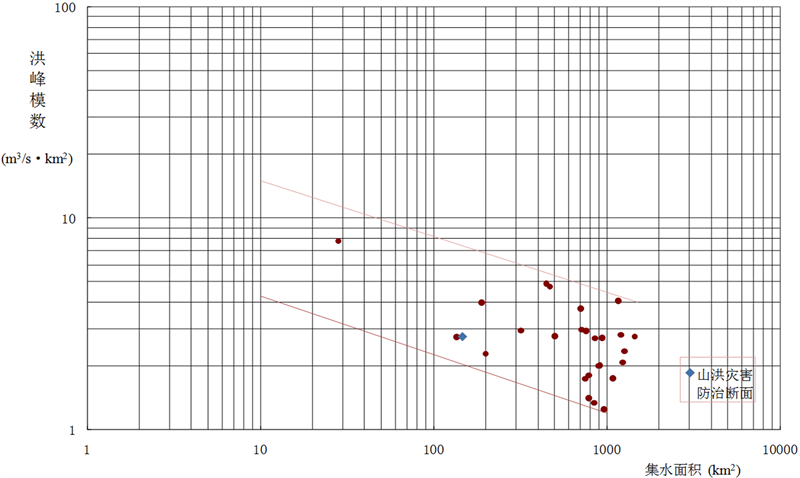﻿ 小流域设计洪水计算方法及应用 Design Flood Estimation Methods and Application in Small Watersheds

Journal of Water Resources Research
Vol.06 No.06(2017), Article ID:22626,8 pages
10.12677/JWRR.2017.66067

Design Flood Estimation Methods and Application in Small Watersheds

Xintian Zhang, Haijin Guo, Jun Shao

Hydrology Bureau, Yangtze River Water Resources Commission, Wuhan HubeiReceived: Oct. 21st, 2017; accepted: Nov. 2nd, 2017; published: Nov. 9th, 2017ABSTRACT

Design floods in small watersheds are important in prevention and control of mountain disaster. Since most of the small rivers in mountain areas are lack of measured flow data, the design flood of small watershed is estimated based on rainfall data. At the same time, rainfall is also an important indicator in prevention and control of mountain disaster. This paper summarizes the main design flood estimation methods in mountainous and small river reaches, and discusses the issues that need to pay attention. The study will provide a reference for the design floods in small watershed for mountain disaster prevention and control.

Keywords:Hydrology, Mountain Disaster, Small Watershed, Design Flood1. 引言

2. 小流域设计洪水计算方法概述

2.1. 推理公式法 

${Q}_{m}=0.278\frac{h}{\tau }F$ (1)

${t}_{c}\ge \tau$ 时，为全面汇流，

${Q}_{m}=0.278\left(\frac{{S}_{P}}{{\tau }^{n}}-\mu \right)F$ (2)

${t}_{c}<\tau$ 时，为部分汇流，

${Q}_{m}=0.278\left(\frac{{S}_{P}{t}_{c}^{1-n}-\mu {t}_{c}}{\tau }\right)F$ (3)

2.2. 单位线法 

$Q\left(t\right)=\underset{0}{\overset{t}{\int }}U\left(t\right)I\left(t-\tau \right)\text{d}t$ (4)

U(t)为系统脉冲响应函数的核函数，即汇流曲线，瞬时入流强度为一个单位时段所形成的出流过程。使用时可将其转换成单位时段响应函数，所谓单位线就是在单位时段内，由流域上时空分布均匀的单位净雨在流域出口断面处形成的地面径流过程线(包括地表径流与壤中流)。单位时段则依流域特性而定，可取l h、3 h、6 h、12 h、24 h等，当时段趋于零时，则相应的单位线称为瞬时单位线。单位净雨量一般取10 mm，也可取l mm。

(1) 经验单位线

${Q}_{t}={\int }_{j=1}^{m}{I}_{j{q}_{i-j+1}}\text{\hspace{0.17em}}\text{\hspace{0.17em}}\left(1\le \left(i-j+1\right)\le m\right),\text{\hspace{0.17em}}i=1,2,3,\cdots ,n;\text{\hspace{0.17em}}j=1,2,3,\cdots ,m$ (5)

(2) 瞬时单位线

J.E.纳希把流域对净雨的调节作用视作等效于n个串联的线性水库的调节作用。一个单位的瞬时入流通过n个水库演进，即可导出瞬时单位线基本公式。

$u\left(0,t\right)=\frac{1}{K\Gamma \left(n\right)}{\left(\frac{t}{k}\right)}^{n-1}{\text{e}}^{-\frac{t}{k}}$ (6)

(3) 综合单位线

① 根据产流计算方法，由流域的设计暴雨推求设计净雨过程； ② 将流域几何特征代入瞬时单位线参数地区综合公式求 ${m}_{1,10}$ 及n(或 ${m}_{2}$ )； ③ 按设计净雨由 ${m}_{1,10}$ 求出设计条件的 ${m}_{1}$ ，并由上一步得到的n求K( $K={m}_{1}/n$ )； ④ 选择时段单位线的净雨时段 $\Delta t$ ，由n、K求时段单位线。 $\Delta t$ 应满足 $\Delta t=\left(\frac{1}{2}~\frac{1}{3}\right){t}_{P}$ 的条件， ${t}_{P}$ 为时段单位线的涨洪历时； ⑤ 由设计净雨过程及时段单位线求得设计地面径流过程； ⑥ 按各省(自治区、直辖市)水文手册、暴雨径流查算图表或暴雨洪水计算等手册或有关设计单位建议的计算方法确定设计条件下的地下径流流量； ⑦ 地面、地下径流过程按相应时刻叠加，即得设计洪水过程。

2.3. 分布式水文模型  

(1) 基于DEM的子流域划分及特征提取：划分子流域，一方面是为了在降雨径流计算过程中充分考虑降雨和下垫面等特征在空间分布的不均匀性，更重要的是为了考虑到山洪防御需要的中、小流域出口等重要断面。最理想的划分方法是将研究流域按自然子流域划分，因为这种划分的计算单元内和单元间的水文过程十分清晰。

(2) 无资料小流域单位线分析：根据径流形成的“粒子说”，流域中水质点汇流时间的概率密度分布函数等价于瞬时单位线，以此为依据分析单位线的基本思路为：首先分析计算流域(包括离散后的形状不规则的计算单元和中、小子流域)各点的径流到达其出口断面的汇流时间；然后推求汇流时间的概率密度分布(横坐标为汇流时间，纵坐标为与其对应的面积占流域面积的比例)——瞬时单位线，然后转换成净雨为1 mm的时段单位线。

(3) 无资料河段马斯京根模型参数：分析马斯京根模型是河道洪水演算的主要方法，目前主要是利用实测水文资料确定其参数，不能用于无实测水文资料河段，这限制了马斯京根模型在分布或半分布式水文模型中的应用。马斯京根模型参数与河段地貌特征以及洪水特征有关，可以通过建立各参数与河段地貌特征间的关系，解决无资料河段马斯京根模型的应用问题，同时，通过建立模型参数与洪水过程间的关系，实现不同洪水过程采用不同的模型参数。

(4) 根据所划分的小流域进行流域水文模型空间关系建模，形成小流域之间的上下游关联；基于DEM数据分析每个小流域的地貌单位线，作为小流域汇流计算的基础；对流域中的水库进行建模，自动分析查找历史洪水资料，并根据历史降雨资料构建数字雨量模型，同时计算某场洪水的前期影响雨量，进行分布式流域产汇流计算、河道演算及水库调蓄演算，对模型演算结果进行分析。

3. 山丘区小流域设计洪水计算中的相关问题探讨

4. 典型实例分析

(1) 设计暴雨

(2) 明流区设计洪水

${Q}_{p.\stackrel{¯}{n}}=0.357\cdot {\gamma }^{0.922}\cdot {f}^{0.125}\cdot {J}^{0.082}\cdot {F}^{0.834}\cdot {\left[C\cdot {K}_{p}\cdot {\stackrel{¯}{H}}_{24}\right]}^{1.23}$ (7)

${\tau }_{p}=0.278\cdot {F}^{0.32}/\left[\gamma \cdot {J}^{0.09}\cdot {f}^{0.13}\cdot {Q}_{p\cdot \stackrel{¯}{n}}^{0.25}\right]$ (8)

(3) 伏流区设计洪水

1) 伏流区地表设计洪水

${Q}_{p.\stackrel{¯}{n}}=0.357\cdot {\gamma }^{0.922}\cdot {f}^{0.360}\cdot {J}^{0.240}\cdot {F}^{0.716}\cdot {\left[C\cdot {K}_{p}\cdot {\stackrel{¯}{H}}_{24}\right]}^{1.23}$ (9)

${\tau }_{p}=0.278\cdot {F}^{0.32}/\left[\gamma \cdot {J}^{0.09}\cdot {f}^{0.13}\cdot {Q}_{p\cdot \stackrel{¯}{n}}^{0.25}\right]$ (10)

2) 经地下河调蓄后的出流过程

(4) 山洪灾害防治断面设计洪水

5. 结论Table 1. Design 24 h rainfall of surface runoff and underground flow in typical watershedTable 2. Design flood peak and volume of surface runoff in typical watershedTable 3. Design flood peak and volume of underground flow in typical watershedTable 4. Design flood peak and volume in typical watershedFigure 1. Design flood hydrographs in typical watershedFigure 2. Flood peak modulus diagram of some hydrological stations in Guizhou Province

Design Flood Estimation Methods and Application in Small Watersheds[J]. 水资源研究, 2017, 06(06): 577-584. http://dx.doi.org/10.12677/JWRR.2017.66067

1. 1. 中国电力工程顾问集团中南电力设计院. 电力工程水文气象计算手册[M]. 武汉: 湖北科学技术出版社, 2011. China Electric Power Engineering Consulting Group Zhongnan Electric Power Design Institute. Handbook of hydrological and meteorological calculations for electric power engineering. Wuhan: Hubei Science and Technology Press, 2011.

2. 2. 季学武, 王俊, 等. 水文分析计算与水资源评价[M]. 北京: 中国水利水电出版社, 2008. JI Xuewu, WANG Jun, et al. Hydrological analysis and water resources evaluation. Beijing: China Water Resources and Hydropower Press, 2008.

3. 3. 郭良, 唐学哲, 孔凡哲. 基于分布式水文模型的山洪灾害预警预报系统研究及应用[J]. 中国水利, 2007(14): 38-41. GUO Liang, TANG Xuezhe and KONG Fanzhe. Studies on mountain flood warning system based on distributed hydrological model and its application. China water resources, 2007(14): 38-41.

4. 4. 罗文兵, 洪林, 时元智. 国内外分布式水文模型研究和应用综述[J]. 中国科技论文在线, 2009. LUO Wenbin, HONG Lin and SHI Yuanzhi. Research and application of distributed hydrological model at home and abroad. Chinese Science and Technology Papers Online, 2009.

5. 5. 中华人民共和国水利行业标准: 水利水电工程设计洪水计算规范[M]. 北京: 中国水利水电出版社, 2006. Regulation calculating design flood of water resources hydropower projects. Beijing: China Water Resources and Hydropower Press, 2006.

6. 6. 水利部长江水利委员会水文局. 水利水电工程设计洪水计算手册[M]. 北京: 水利电力出版社, 1995. Hydrology Bureau, Yangtze River Water Resources Commission, Ministry of Water Resources. Water resources and hydropower engineering design flood calculation manual. Beijing: Water Resources and Hydropower Press, 1995.